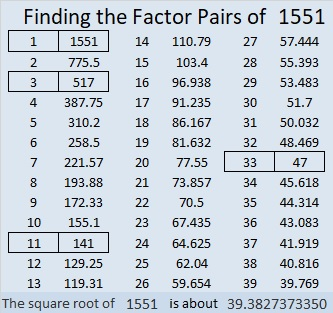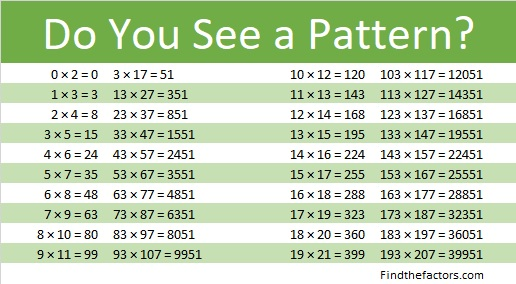# 1551 Two Straight Lines

Contents

### Today’s Puzzle:

The clues in this level 4 puzzle form two straight lines, and most of the logic needed to solve it is rather straightforward. Can you find the factors from 1 to 10 without getting twisted up?### Factors of 1551:

1+5 = 6, a multiple of 3, so 1551 is divisible by 3.
Since 1551 is a palindrome with an even number of digits, it is also divisible by 11.

• 1551 is a composite number.
• Prime factorization: 1551 = 3 × 11 × 47.
• 1551 has no exponents greater than 1 in its prime factorization, so √1551 cannot be simplified.
• The exponents in the prime factorization are 1, 1, and 1. Adding one to each exponent and multiplying we get (1 + 1)(1 + 1)(1 + 1) = 2 × 2 × 2 = 8. Therefore 1551 has exactly 8 factors.
• The factors of 1551 are outlined with their factor pair partners in the graphic below.### A Little More about the Number 1551:

Did you notice how many of 1551’s factors are palindromes?

1551 is the difference of two squares in four different ways:
776² – 775² = 1551,
260² – 257² = 1551,
76² – 65² = 1551, and
40² – 7² = 1551. (Yes, we are just 7² or 49 numbers away from 1600, the next perfect square!)

1551 is in this cool pattern:This site uses Akismet to reduce spam. Learn how your comment data is processed.